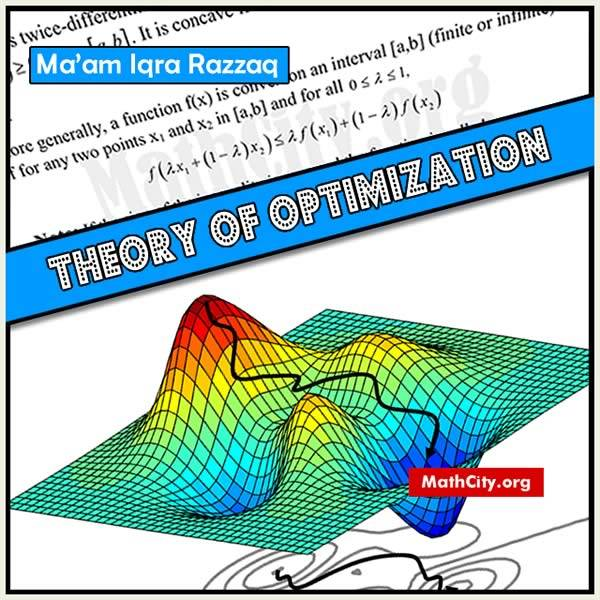# Theory of Optimization by Ma'am Iqra RazzaqThese notes are provided and composed by Mr. Muzammil Tanveer. We are really very thankful to him for providing these notes and appreciates his effort to publish these notes on MathCity.org. These notes are based on the lectures by Ma'am Iqra Razzaq.

Optimization theory is an area of mathematics concerned with determining the optimal solutions to issues involving the minimizing or maximization of a real function. It covers a wide range of minimization and optimization topics, including calculus of variations, control theory, game theory, and linear programming, among others. Numerous formulations and areas of applied mathematics can benefit from the use of optimization theory. A set of design parameters that have an impact on the objective function must typically be defined along with an objective function that has to be maximized or minimized.

 Name Theory of Optimization Mr. Muzammil Tanveer 110 pages PDF (see Software section for PDF Reader) 835 kB
• Introduction
• Formal definition
• Status of Optimization
• Flow chart of Modeling process
• Requirement of Optimization Algorithm
• Types of Optimization
• Constraints
• Convex Function
• Convex Optimization Problem
• Matrix form of equations
• Hessian matrix method
• Unconstraint Optimization problems
• Principle minor diagonal
• Newton Raphson Method (For unconstraint’s Optimization)
• Non-linear programming
• Local and Global optima
• Results from Calculus
• Three-point interval search
• Fibonacci Search method
• Mean Search or Golden Mean search or Golden section search method
• The method of steepest Ascent